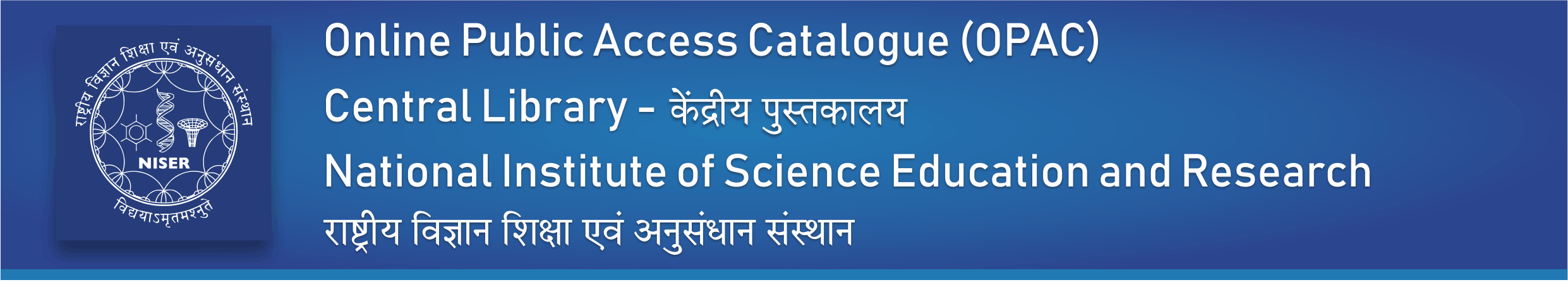Image from Coce
Normal view

# Introduction to numerical geodynamic modelling

Material type:TextLanguage: English Publication details: 2019 Edition: 2nd edDescription: xiii, 471p. hbISBN: 9781107143142Subject(s): GEODYNAMICS | GEOLOGY | GEOPHYSICSDDC classification: 551.2 Summary: This hands-on introduction to numerical geodynamic modelling provides a solid grounding in the necessary mathematical theory and techniques, including continuum mechanics and partial differential equations, before introducing key numerical modelling methods and applications. Fully updated, this second edition includes four completely new chapters covering the most recent advances in modelling inertial processes, seismic cycles and fluid-solid interactions, and the development of adaptive mesh refinement algorithms. Many well-documented, state-of-the-art visco-elasto-plastic 2D models are presented, which allow robust modelling of key geodynamic processes. Requiring only minimal prerequisite mathematical training, and featuring over sixty practical exercises and ninety MATLAB® examples, this user-friendly resource encourages experimentation with geodynamic models. It is an ideal introduction for advanced courses and can be used as a self-study aid for graduates seeking to master geodynamic modelling for their own research projects. • Provides an optimal study path based on the author's extensive experience of teaching the topic • Continuum mechanics and numerical modelling are explained in simple terms, making this an accessible introductory text for graduate students • A uniform and simple numerical method is used enabling the reader to understand modelling of many complex problems using the same simple method • Many analytical and programming exercises are proposed with online MATLAB examples provided, allowing students to test their knowledge
Tags from this library: No tags from this library for this title.
Star ratingsAverage rating: 3.0 (1 votes)
Holdings
Item type Current library Call number Status Date due BarcodeBook NISER LIBRARY
551.2 GER-I (Browse shelf(Opens below)) Available 24655Book NISER LIBRARY
551.2 GER-I (Browse shelf(Opens below)) Available 24295Book NISER LIBRARY
551.2 GER-I (Browse shelf(Opens below)) Checked out to Tiyasha Basu (22127002) 28/09/2023 24296Book NISER LIBRARY
551.2 GER-I (Browse shelf(Opens below)) Checked out to Souvik Daripa (23127003) 01/10/2023 23236

1. The continuity equation
2. Density and gravity
3. Numerical solutions of partial differential equations
4. Stress and strain
5. The momentum equation
6. Viscous rheology of rocks
7. Numerical solutions of the momentum and continuity equations
8. The advection equation and marker-in-cell method
9. The heat conservation equation
10. Numerical solution of the heat conservation equation
11. 2D thermomechanical code structure
12. Elasticity and plasticity
13. 2D implementation of visco-elasto-plasticity
14. 2D thermomechanical modelling of inertial processes
15. Seismo-thermomechanical modelling
16. Hydro-thermomechanical modelling
18. The multigrid method
19. Programming of 3D problems
20. Numerical benchmarks
21. Design of 2D numerical geodynamic models
Epilogue: outlook
Appendix: MATLAB® program examples
References
Index

This hands-on introduction to numerical geodynamic modelling provides a solid grounding in the necessary mathematical theory and techniques, including continuum mechanics and partial differential equations, before introducing key numerical modelling methods and applications. Fully updated, this second edition includes four completely new chapters covering the most recent advances in modelling inertial processes, seismic cycles and fluid-solid interactions, and the development of adaptive mesh refinement algorithms. Many well-documented, state-of-the-art visco-elasto-plastic 2D models are presented, which allow robust modelling of key geodynamic processes. Requiring only minimal prerequisite mathematical training, and featuring over sixty practical exercises and ninety MATLAB® examples, this user-friendly resource encourages experimentation with geodynamic models. It is an ideal introduction for advanced courses and can be used as a self-study aid for graduates seeking to master geodynamic modelling for their own research projects.
• Provides an optimal study path based on the author's extensive experience of teaching the topic
• Continuum mechanics and numerical modelling are explained in simple terms, making this an accessible introductory text for graduate students
• A uniform and simple numerical method is used enabling the reader to understand modelling of many complex problems using the same simple method
• Many analytical and programming exercises are proposed with online MATLAB examples provided, allowing students to test their knowledge

There are no comments on this title.